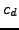Next: *FREQUENCY Up: Input deck format Previous: *FLUID CONSTANTS   Contents

## *FLUID SECTION

Keyword type: model definition

This option is used to assign material properties to network element sets. The parameters ELSET and MATERIAL are required, the parameters TYPE and OIL are optional. The parameter ELSET defines the network element set to which the material specified by the parameter MATERIAL applies.

The parameter TYPE is only necessary in fluid dynamic networks in which the pressure and/or the mass flow are unknown in at least one node. In that case, the type of fluid section must be selected from the list in section 6.2.29 and the appropriate constants describing the section must be specified in the line(s) underneath the *FLUID SECTION keyword card, eight per line, except for the last line which can contain less.

Finally, the parameter OIL defines the material parameters used in two-phase flow in gas pipes, restrictors and branches. Its argument must be the name of a material defined using the *MATERIAL card.

First line:

• *FLUID SECTION
• Enter any needed parameters.

Following line (only necessary if TYPE was used):

• First constant
• Second constant
• etc (maximum eight constants on this line)
Repeat this line if more than eight constants are needed to describe the fluid section.

```Example:

*FLUID SECTION,MATERIAL=NITROGEN,ELSET=Eall
```

assigns material NITROGEN to all elements in (element) set Eall.

```Example:

*FLUID SECTION,MATERIAL=AIR,ELSET=Eall,TYPE=ORIFICE_PK_MS
3.14,0.1,2.,0.01,0.1
```

assigns material AIR to all elements in set Eall. The type of fluid section is an orifice with thecoefficient calculated following the formulas by Parker and Kercher , modified for the influence of the rotational velocity by McGreehan and Schotsch . The area of the orifice is 3.14, the length is 0.1, the diameter is 2., the inlet corner radius is 0.01 and the pipe diameter ratio is 0.1.

Example files: furnace, beamhtfc, branch1.Next: *FREQUENCY Up: Input deck format Previous: *FLUID CONSTANTS   Contents
guido dhondt 2014-03-02